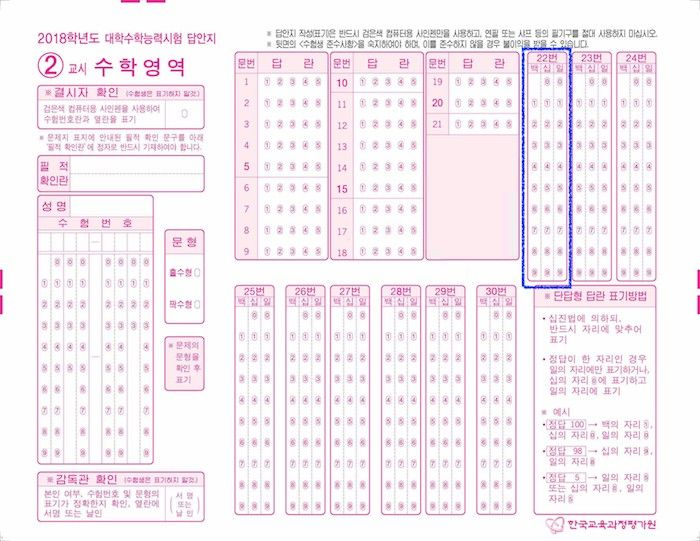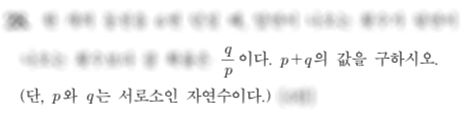시간 제한메모리 제한제출정답맞힌 사람정답 비율
1 초 (추가 시간 없음) 1024 MB185989461.039%

## 문제

About 44 days are left before College Scholastic Ability Test is held. This exam aims to measure students' achievement of National Curriculum standards and scholastic ability required for college education. (http://www.kice.re.kr/sub/info.do?m=0205&s=english)

One of the subjects covered by this test is Mathematics, which consists of 21 multiple choice questions and 9 short-answer questions. The answer of each short-answer question is guaranteed to be a unique positive integer below 1000, as you can see from the answer sheet below.However, the organizers might want to give students short-answer questions with non-integer answers, such as $2\sqrt{3}$ or $\frac{5}{3}$. Usually, the workaround is to write the answer in a canonical form, and then sum up all the integers inside that form and ask students to write that number instead.

In particular, when the answer is a positive rational number $\frac{a}{b}$, the organizers usually ask students to reduce it and sum up the numerator and the denominator of the reduced fraction. For example, when the answer is $\frac{18}{10}$, the student should reduce it to $\frac{9}{5}$ and write the final answer as $9 + 5 = 14$.However, when the answer is $\frac{521}{500}$, the reduced fraction is also $\frac{521}{500}$, so the student should write the final answer as $521 + 500 = 1021$. But this shouldn't happen, since all the answers for the short-answer questions are below 1000. To avoid this situation, the organizers should make sure that after reducing the fraction, the sum of the numerator and the denominator shouldn't exceed $999$. Let's call such fractions as Suneung Fractions. For example, $\frac{1996}{2}$ and $\frac{18}{10}$ are Suneung fractions, while $\frac{1998}{2}$ and $\frac{521}{500}$ are not.

Suppose that, this year, one of the organizers wrote a problem, and the answer to that problem is $\frac{x}{y}$. Since the problem is not finalized yet, the only thing we know is $A \le x \le B$ and $C \le y \le D$ holds, for given $A, B, C, D$. The organizers want to know, among all the pairs $(x, y)$, how many of $\frac{x}{y}$ is a Suneung fraction. Write a program that counts this number.

## 입력

The first and only line contains four space-separated integers $A, B, C$ and $D$ ($1 \le A \le B \le 10^{12}$, $1 \le C \le D \le 10^{12}$)

## 출력

Print the number of integral pairs $(x,\ y)$ ($A \le x \le B$, $C \le y \le D$), where $\frac{x}{y}$ is a Suneung fraction.

## 예제 입력 1

5 8 3 6


## 예제 출력 1

16


## 예제 입력 2

2018 2019 2018 2019


## 예제 출력 2

2